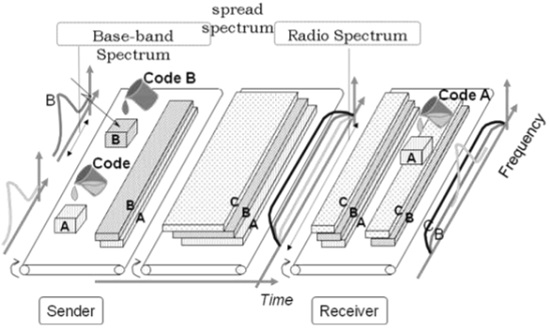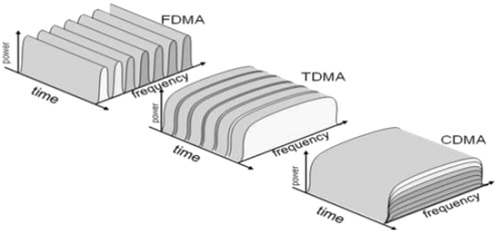# CDMA - Technology

Code Division Multiple Access (CDMA) is a sort of multiplexing that facilitates various signals to occupy a single transmission channel. It optimizes the use of available bandwidth. The technology is commonly used in ultra-high-frequency (UHF) cellular telephone systems, bands ranging between the 800-MHz and 1.9-GHz.

## CDMA Overview

Code Division Multiple Access system is very different from time and frequency multiplexing. In this system, a user has access to the whole bandwidth for the entire duration. The basic principle is that different CDMA codes are used to distinguish among the different users.

Techniques generally used are direct sequence spread spectrum modulation (DS-CDMA), frequency hopping or mixed CDMA detection (JDCDMA). Here, a signal is generated which extends over a wide bandwidth. A code called spreading code is used to perform this action. Using a group of codes, which are orthogonal to each other, it is possible to select a signal with a given code in the presence of many other signals with different orthogonal codes.

## How Does CDMA Work?

CDMA allows up to 61 concurrent users in a 1.2288 MHz channel by processing each voice packet with two PN codes. There are 64 Walsh codes available to differentiate between calls and theoretical limits. Operational limits and quality issues will reduce the maximum number of calls somewhat lower than this value.

In fact, many different "signals" baseband with different spreading codes can be modulated on the same carrier to allow many different users to be supported. Using different orthogonal codes, interference between the signals is minimal. Conversely, when signals are received from several mobile stations, the base station is capable of isolating each as they have different orthogonal spreading codes.

The following figure shows the technicality of the CDMA system. During the propagation, we mixed the signals of all users, but by that you use the same code as the code that was used at the time of sending the receiving side. You can take out only the signal of each user.## CDMA Capacity

The factors deciding the CDMA capacity are −

• Processing Gain
• Signal to Noise Ratio
• Voice Activity Factor
• Frequency Reuse Efficiency

Capacity in CDMA is soft, CDMA has all users on each frequency and users are separated by code. This means, CDMA operates in the presence of noise and interference.

In addition, neighboring cells use the same frequencies, which means no re-use. So, CDMA capacity calculations should be very simple. No code channel in a cell, multiplied by no cell. But it is not that simple. Although not available code channels are 64, it may not be possible to use a single time, since the CDMA frequency is the same.

## Centralized Methods

• The band used in CDMA is 824 MHz to 894 MHz (50 MHz + 20 MHz separation).
• Frequency channel is divided into code channels.
• 1.25 MHz of FDMA channel is divided into 64 code channels.

## Processing Gain

CDMA is a spread spectrum technique. Each data bit is spread by a code sequence. This means, energy per bit is also increased. This means that we get a gain of this.

P (gain) = 10log (W/R)

R is Data Rate

For CDMA P (gain) = 10 log (1228800/9600) = 21dB

This is a gain factor and the actual data propagation rate. On an average, a typical transmission condition requires a signal to the noise ratio of 7 dB for the adequate quality of voice.

Translated into a ratio, signal must be five times stronger than noise.

Actual processing gain = P (gain) - SNR

= 21 – 7 = 14dB

CDMA uses variable rate coder

The Voice Activity Factor of 0.4 is considered = -4dB.

Hence, CDMA has 100% frequency reuse. Use of same frequency in surrounding cells causes some additional interference.

In CDMA frequency, reuse efficiency is 0.67 (70% eff.) = -1.73dB

CDMA has a soft capacity. The greater the number of codes, the more the number of users. It has the following advantages −

• CDMA requires a tight power control, as it suffers from near-far effect. In other words, a user near the base station transmitting with the same power will drown the signal latter. All signals must have more or less equal power at the receiver

• Rake receivers can be used to improve signal reception. Delayed versions of time (a chip or later) of the signal (multipath signals) can be collected and used to make decisions at the bit level.

• Flexible transfer may be used. Mobile base stations can switch without changing operator. Two base stations receive mobile signal and the mobile receives signals from the two base stations.

• Transmission Burst − reduces interference.